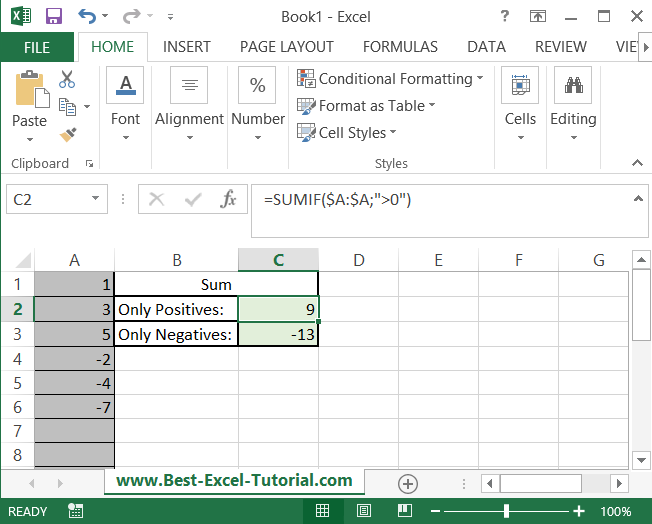#### How to Sum only Positive / Negative Numbers in Excel

In this tutorial you will teach yourself how to sum only positive or only negative numbers from your range.

## Sumif formula

Suppose that you have some values in A column. Your task is to sum positive only or negative only values. To do that just use one of these formulas:

• For positives: =SUMIF(\$A:\$A,”>0″)
• For negatives: =SUMIF(\$A:\$A,”<0″)This is how to sum only positive numbers in Excel.

## Sumifs formula

Alternatively, you can use SUMIFS Excel function to add only possitive values.

The formula in our example will look as follows: =SUMIFS(A1:A6,A1:A6,”>0″)

The output of Sumifs function will be exactly the same as in the first example.

Sumifs function offers one additional advantage. After the comma you are able to add one or more conditions.

=SUMIFS(A1:A6,A1:A6,”>0″,B1:B6,”tom”)

With such an example only positive values in given range are calculated as soon as there is a “tom” string in Column B.

Isn’t it easy? Excel works great when you can create such formulas. In case you needed to sum positive integers or only negative values you may use above formula.

## Conditional Sum

This method allows you to sum cells based on a conditional formula. To sum only positive numbers, follow these steps:

1. In a blank cell, enter the formula =SUM(IF(range>0, range, 0)).
2. Replace “range” with the range of cells that you want to sum.
3. Press “Enter” to get the sum of only the positive numbers in the specified range.

Note: In all the above methods, you can replace the positive number condition (>0) with a negative number condition (<0) to sum only negative numbers.